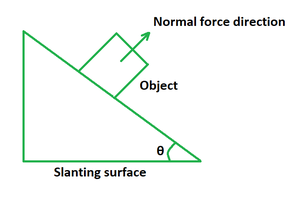Open in App
Not now

# Normal Force Formula

• Last Updated : 11 May, 2022

The normal force is defined as the force exerted by any surface on another object. When an object is at rest, the net force exerted on it is equal to zero. A normal force cannot be applied on two surfaces that are not connected to one another. It can be interpreted as a component of the force that is vertical to any contact surface. It determines how much force the body delivers to the ground. The normal force is equal to the object’s weight only if the object’s rate of velocity change is negative, which means it is decelerating.Formula

The value of normal force depends on where the object is placed with respect to the other object. When an object is going to fall, the position in which the object falls on the ground determines the value of normal force. The normal force is denoted by the symbol FN. Its unit of measurement is Newtons (N) and the dimensional formula is given by [M1L1T-2].

If a body is resting on a flat force, the normal force equals the value of the gravitational weight, that is, mg.

FN = mg

where,

FN is the normal force,

m is the mass of resting object,

g is the acceleration due to gravity.

If a body is sliding down from a slant surface at some angle, the value of normal force is gravitational weight added by an extra force of F sin θ. In this case, the normal force is greater than the weight of the object.

FN = mg + F sin θ

Where,

FN is the normal force,

m is the mass of sliding object,

g is the acceleration due to gravity,

θ is the inclination angle.

If the force is acting on a body in an upward direction, the value of normal force is gravitational weight decremented by a force of F sin θ. In this case, the net normal force is less than the weight of the object.

FN = mg – F sin θ

where,

FN is the normal force,

m is the mass of sliding object,

g is the acceleration due to gravity,

θ is the inclination angle.

If a body is placed on an inclined plane, the normal force FN equals the product of gravitational weight and cosine of inclination angle.

FN = mg cos θ

where,

FN is the normal force,

m is the mass of sliding object,

g is the acceleration due to gravity,

θ is the inclination angle.

### Sample Problems

Problem 1. An object of mass 2 kg is resting on a table. Calculate the normal force being exerted on it.

Solution:

We have,

m = 2

g = 9.8

Using the formula we get,

FN = mg

= 2 (9.8)

= 19.6 N

Problem 2. An object is resting on a table with a force of 39.2 N. Calculate the normal force being exerted on it.

Solution:

We have,

F = 39.2

g = 9.8

Using the formula we get,

FN = mg

=> m = F/g

=> m = 39.2/9.8

=> m = 4 kg

Problem 3. An object of mass of 10 kg is sliding down with a force of 200 N from a slant surface at an angle of 30°. Calculate the normal force being exerted on it.

Solution:

We have,

F = 200

m = 10

g = 9.8

θ = 30°

Using the formula we get,

FN = mg + F sin θ

= 10 (9.8) + 200 sin 30°

= 98 + 200 (1/2)

= 98 + 100

= 198 N

Problem 4. An object of mass 20 kg is sliding down with a force of 400 N from a slant surface at an angle of 30°. Calculate the normal force being exerted on it.

Solution:

We have,

F = 400

m = 20

g = 9.8

θ = 30°

Using the formula we get,

FN = mg + F sin θ

= 20 (9.8) + 400 sin 30°

= 196 + 400 (1/2)

= 196 + 200

= 396 N

Problem 5. An object of mass of 15 kg is placed on a slant surface at an angle of 30°. Calculate the normal force being exerted on it if force is acting upwards with a value of 100 N.

Solution:

We have,

F = 100

m = 15

g = 9.8

θ = 30°

Using the formula we get,

FN = mg – F sin θ

= 15 (9.8) – 100 sin 30°

= 147 – 100 (1/2)

= 147 – 50

= 97 N

Problem 6. An object of mass 5 kg is placed on a slant surface at an angle of 60°. Calculate the normal force being exerted on it at any point.

Solution:

We have,

m = 5

g = 9.8

θ = 60°

Using the formula we get,

FN = mg cos θ

= 5 (9.8) (cos 60°)

= 49/2

= 24.5 N

Problem 7. An object is placed on a slant surface at an angle 60°. Calculate its mass if the normal force being exerted on it is 400 N.

Solution:

We have,

FN = 400,

θ = 60°

Using the formula we get,

FN = mg cos θ

m = F/(g cos θ)

= 400/ (9.8 × cos 60°)

= 400/4.9

= 81.63 N

My Personal Notes arrow_drop_up